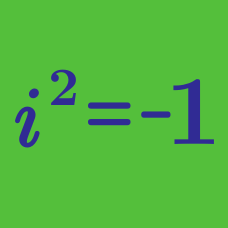Algebra

# Complex Numbers - Factoring Polynomials

A quadratic polynomial with real coefficients has $19 + 8 i$ as a root. If the other root has the form $a + bi$, where $a$ and $b$ are real numbers, what is the value of $a + b$?

If $x (x-4-9i)(x-a+bi) = x^3 -8x^2+97x,$ what is $a + b$ ?

Details and assumptions

$i$ is the the imaginary unit, defined by $i^2 = -1$.

Suppose that $7x^2-14x+182=c(x-a-bi)(x-a+bi),$ where $i$ is the imaginary number that satisfies $i^2=-1$ and $b > 0.$ What is the value of $a+b+c$?

Suppose that \begin{aligned} & x^4+6x^3+17x^2-6x-18 \\ &= (x+a)(x-a)(x+b+ci)(x+b-ci), \end{aligned} where $i$ is the imaginary number that satisfies $i^2=-1$. What is the value of $a+b+c$?

Details and assumptions

Assume $a > 0$ and $c > 0$.

It is given that the cubic polynomial $f(x)$ has real coefficients, and $f(x) = 0$ has a complex root $28 - 15 i$. If the sum of all the complex, non-real roots of $f(x) = 0$ can be written as $a + bi$, what is the value of $a + b$?

×